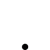# 自動下單

•   272
• 最後發表   東尼  4 週前

工程師 您好:

1. 想用自動交易控制停損   例如: close < 買進價5% 賠5%就自動執行停損賣出

(可指定時間或收盤前嗎? 例如: 1點或1:20  因盤中有時只是急殺)

2. 如果有庫存股 比如 2330 已經賠7%  可以排除指定的股票  暫不執行停損嗎?

XQ小幫手 發文於   2021/08/04

Hello 東尼,

https://xshelp.xq.com.tw/XSHelp/?HelpName=FilledAvgPrice&group=TRANSACTIONFUNC

condition1 = currenttime >= 130000; //時間大於13:00

condition2 = close <= (FilledAvgPrice * 0.95);  //收盤價小於進場價格的95%

if condition1 and condition2 and position <> 0 then setposition(0, market);  //如果達成上面兩個條件，且部位不為0的話清空部位

https://xshelp.xq.com.tw/XSHelp/?HelpName=Symbol&group=FIELDFUNC

condition1 = currenttime >= 130000; //時間大於13:00

condition2 = close <= (FilledAvgPrice * 0.95);  //收盤價小於進場價格的95%

if condition1 and condition2 //如果達成上面兩個條件

and position <> 0 and symbol <> "2330.TW" //部位不為0且商品不為2330.TW

then setposition(0, market);  //清空部位

1.請問完整腳本這樣寫對嗎?  停損/停利一起寫對嗎?   要放在"交易"類腳本對嗎?

2.腳本若正確 經過設定帳正確後  就會自行去抓指定帳號庫存 是嘛?

3.腳本執行頻率需要(不要)設定?

4.因剛開始使用 如果只想在達到-停損或停利條件時 只要出清一半(1/2)部位 請問怎改?

---------------------------------------------------------------------------------------------------

if filled >0 then  begin

condition1 = currenttime >= 130000;  // 時間大於13:00 後決定

condition2 = close <= (FilledAvgPrice * 0.95); //收盤價小於進場價格的95%

condition3 = close >= (FilledAvgPrice * 1.10); //收盤價大於進場價格的 10%

if condition1 and condition2 //如果達成上面1&2兩個條件- 停損

if condition1 and condition3 //如果達成上面1&3兩個條件- 停利

and position <> 0 and symbol <> "2330.TW" //部位不為0且商品不為2330.TW

then setposition(0, market); //清空部位

-------------------------------------------------------------------------------

5.如果要用20日均線停損時  是否改成指定這個件就好了

condition1 = currenttime >= 130000;  // 時間大於13:00 後決定

//condition2 = close <= (FilledAvgPrice * 0.95); //收盤價小於進場價格的95%

//condition3 = close >= (FilledAvgPrice * 1.10); //收盤價大於進場價格的 10%

condition4 = close <= average(getfield("收盤價", "D"),20) ;//20日均價

if condition1 and condition4 //如果達成上面1&4兩個條件-

then setposition(0, market); //清空部位

XQ小幫手 發文於   2021/08/18

Hello 東尼,

1.

if filled > 0 and position > 0 then begin

condition1 = currenttime >= 130000;  // 時間大於13:00 後決定

condition2 = close <= (FilledAvgPrice * 0.95); //收盤價小於進場價格的 95%

condition3 = close >= (FilledAvgPrice * 1.10); //收盤價大於進場價格的 110%

condition4 = symbol <> "2330.TW";  //商品不為2330.TW

if condition1 and (condition2 or condition3) and condition4 then setposition(0, market); //如果達成上面1&(2 or 3)&4條件-市價出場

end;

2.

3.

4.

input: qty(4, "初始持有張數");

if filled > floor(qty/2) and position > floor(qty/2) then begin

condition1 = currenttime >= 130000;  // 時間大於13:00 後決定

condition2 = close <= (FilledAvgPrice * 0.95); //收盤價小於進場價格的 95%

condition3 = close >= (FilledAvgPrice * 1.10); //收盤價大於進場價格的 110%

condition4 = symbol <> "2330.TW";  //商品不為2330.TW

if condition1 and (condition2 or condition3) and condition4 then setposition(floor(qty/2), market); //如果達成上面1&(2 or 3)&4條件-市價出場

end;

5.

1.若股價到設定的停損/停利價格時  如果要指定用  市價  內盤價  外盤價 或限定價格的方式賣出  該如何定義?

2.如果早上10點買一檔股票100元  到13:10分時股價跌到94元  該腳本會執行動作嗎?  或該如何做?

如果庫存是融資 或是當沖該注意甚麼嗎?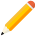發表新主題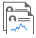我的發文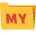我的收藏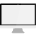分類